Courses

NCERT Solutions (Ex - 11.3, 11.4) - Mensuration Notes - Class 8

Class 8: NCERT Solutions (Ex - 11.3, 11.4) - Mensuration Notes - Class 8

The document NCERT Solutions (Ex - 11.3, 11.4) - Mensuration Notes - Class 8 is a part of Class 8 category.
All you need of Class 8 at this link: Class 8

Exercise 11.3

Question 1:

There are two cuboidal boxes as shown in the adjoining figure. Which box requires the lesser amount of material to make?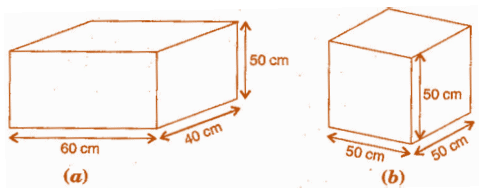(b) Given:  Length of cuboidaJ box (/) =60 cm

Breadth of cuboidal box (b) = 40 cm

Height of cuboidal box (h)= 50 cm

Total surface area of cuboidal box = 2(Ib + bh + hl)

= 2 [60x40 + 40x50 + 50 x 60)

= 2 [2400 + 2000 + 3000]

= 2 x 7400 = 14800 cm2

(b) Given:  Length of cuboidal box (/) =50 cm

Breadth of cuboidal box (b) = 50 cm

Height of cuboidal box (h) = 50 cm

Total surface area of cuboidaJ box = 2(Ib + bh + hl)

= 2 [50x50 + 50x50 + 50 x 50]

= 2 [2500 + 2500 + 2500]

= 2x7500 = 15000 cm2

Hence, the cuboidal box (a) requires the lesser amount of material to make, since surface area of box (a) is less than that of box (b).

Question 2:

A suitcase with measures 80 cm x 48 cm x 24 cm is to be covered with a tarpaulin cloth. How many meters of tarpaulin of width 96 cm is required to cover 100 such suitcases?

Given: Length of suitcase box (/) =80 cm,

Breadth of suitcase box (b) = 48 cm

And Height of cuboidal box (h) = 24 cm

Total surface area of suitcase box = 2 (lb + hh + hl)

= 2 [80 x 48 + 48 x 24 + 24 x 80]

= 2 [3840 + 1152 + 1920]

= 2 x 6912 = 13824 cm2

Area of Tarpaulin cloth = Surface area of suitcase

=>       Ixb =13824

=>       / x 96 = 13824

=> l = 13824/96 = 144 cm

Required tarpaulin for 100 suitcase s = 144 x 100 = 14400 cm = 144 m

Hence, the tarpaulin cloth required to cover 100 suitcases is 144 m.

Question 3:

Find the side of a cube whose surface area id 600 cm2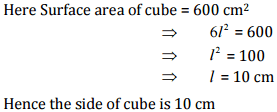Question 4:

Rukshar painted the outside of the cabinet of measure 1 m x 2 m x 1.5 m. How much surface area did she cover if she painted all except the bottom of the cabinet?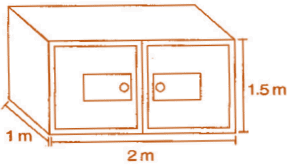Here,

Length of cabinet (1) = 2 m,

Breadth of cabinet (b) = 1 m

And Height of cabinet (h) = 1.5 m

Surface area of cabinet = lb + 2(bh + hi)

= 2x1 + 2 (1 x 1.5 +1.5 x 2)

= 2 + 2 [1.5 + 3.0)

= 2 + 9.0

= 11m2

Hence, the required surface area of cabinet is 11 m2.

Question 5:

Daniel is paining the walls and ceiling of a cuboidal hall with length, breadth and height of 15 m, 10 m and 7 m respectively. From each can of paint 100 m2 of area is painted. How many cans of paint will she need to paint the room?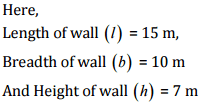Total Surface area of classroom = lb + 2(bh + hl)

= 15x10 + 2 [10x7 + 7x15)

= 150 + 2 [70 + 105)

= 150 + 350

= 500 m2

Now, Required numbers of cans = area of hall/area of one can = 500/100 = 5 cans

hence, 5 cans are required to paint the room

Question 6:

Describe how the two figures below are alike and how they are different. Which box has larger lateral surface area?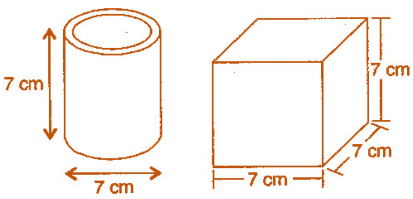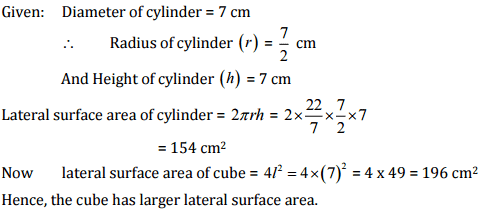Question 7:

A closed cylindrical tank of radius 7 m and height 3 m is made from a sheet of metal. How much sheet of metal is required?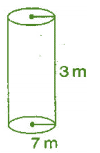Given:  Radius of cylindrical tank (r) = 7 m

Height of cylindrical tank (h) = 3 m

Total surface area of cylindrical tank = 2 πr(h+r)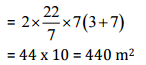Hence, 440 m2 metal sheet is required.

Question 8:

The lateral surface area of a hollow cylinder is 4224 cm2. It is cut along its height and formed a rectangular sheet of width 33 cm. Find the perimeter of rectangular sheet?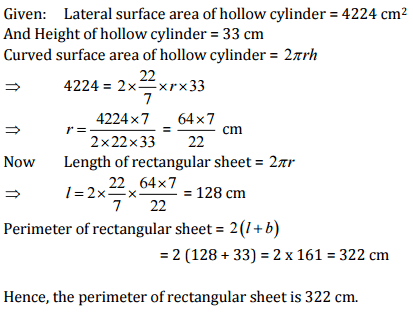Question 9:

A road roller takes 750 complete revolutions to move once over to level a road. Find the area of the road if the diameter of a road roller is 84 cm and length 1 m.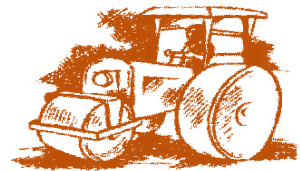Given:   Diameter of road roller = 84 cm

Length of road roller (h) = 1 m = 100 cm

Curved surface area of road roller = 2πrh = 2 x 22/7 x 42 x 1600 = 26400 cm2

Area covered by road roller in 750 revolutions = 26400 x 750

= 1,98,00,000 cm2

= 1980 m2 [ ∵1 m= 10,000 cm2)

Hence, the area of the road is 1980 m2.

Question 10:

A company packages its milk powder in cylindrical container whose base has a diameter of 14 cm and height 20 cm. Company places a label around the surface of the container (as shown in figure). If the label is placed 2 cm from top and bottom, what is the area of the label?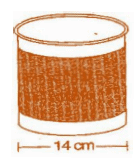Given: Diameter of cylindrical container = 14 cm

∴ Radius of cylindrical container (r) = d/2 = 14/2 = 7cm

Height of cylindrical container = 20 cm

Height of the label (h)= 20 - 2 - 2 = 16 cm

Curved surface area of label = 2πrh = 2 x 22/7 x 7x 16 = 704 cm2

Hence, the area of the label of 704 cm2.

Exercise 11.4

Question 1:

Given a cylindrical tank, in which situation will you find surface are and in which situation volume.

(a) To find how much it can hold.

(b) Number of cement bags required to plaster it.

(c) To find the number of smaller tanks that can be filled with water from it.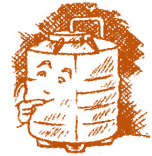We find area when a region covered by a boundary, such as outer and inner surface area of a cylinder, a cone, a sphere and surface of wall or floor.

When the amount of space occupied by an object such as water, milk, coffee, tea, etc., then we have to find out volume of the object.

(a) Volume

(b) Surface area

(c) Volume

Question 2:

Diameter of cylinder A is 7 cm and the height is 14 cm. Diameter of cylinder B is 14 cm and height is 7 cm. Without doing any calculations can you suggest whose volume is greater? Verify it by finding the volume of both the cylinders. Check whether the cylinder with greater volume also has greater surface area.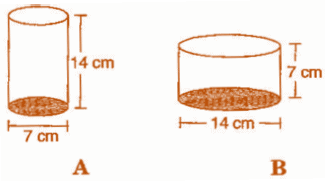Yes, we can say that volume of cylinder B is greater, since radius of cylinder B is greater than that of cylinder A (and square of radius gives more value than previous).

Diameter of cylinder A = 7 cm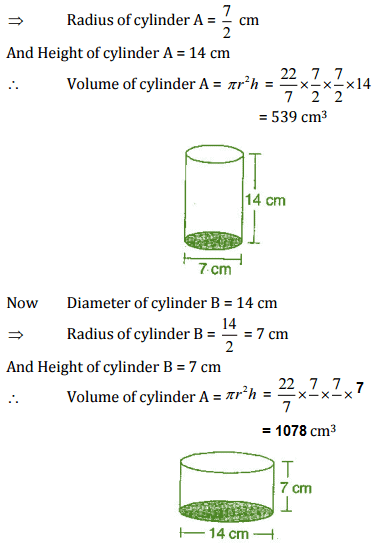Total Surface area of cylinder A = πr(2h+r)Total Surface area of cylinder B = πr(2h+r)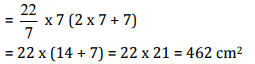Yes, Cylinder with greater volume also has greater surface area.

Question 3:

Find the height of a cuboid whose base area is 180 cm2 and volume is 900 cm

Given:  Base area of cuboid = 180 cm2 and

Volume of cuboid = 900 cm3

We know that,

Volume of cuboid = Ixbxh

=> 900 = 180 xh     [Base area = /x b - 180(given)]

=> h = 900/180 = 5m

Hence, the height of cuboid is 5 m.

Question 4:

A cuboid is of dimensions 60 cm x 54 cm x 30 cm. How many small cubes with side 6 cm can be placed in the given cuboid?

Given:

Length of cuboid (/) = 60cm,

Breadth of cuboid ( 6) = 54 cm and,

Height of cuboid (h) = 30 cm

We know that, Volume of cuboid = Ixbxh = 60 x 54 x 30 cm3

And

Volume of cube = (Side)3 = 6x6x6 cm3

Number of small cubes =Hence, the required cubes are 450

Question 5:

Find the height of the cylinder whose volume if 1.54 m3 and diameter of the base is 140 cm.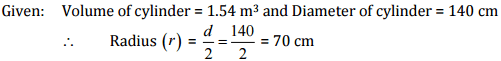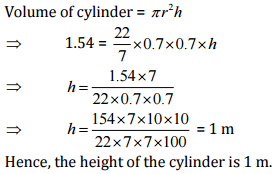Question 6:

A milk tank is in the form of cylinder whose radius is 1.5 m and length is 7 m. Find the quantity of milk in liters that can be stored in the tank.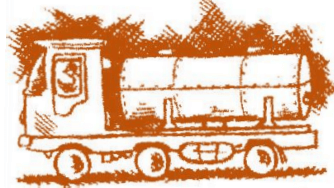Given: Radius of cylindrical tank (r) = 1.5 m

And Height of cylindrical tank (h) = 7 m

Volume of cylindrical tank = πr2h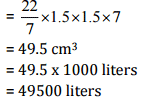Hence, the required quantity of milk is 49500 lt.

Question 7:

If each edge of a cube is doubled,

(i) how many times will its surface area increase?

(ii) how many times will its volume increase?

(i)

Let the edge of cube be 1.

Since, Surface area of the cube (A) = 6l2

When edge of cube is doubled, then

Surface area of the cube (A') = 6 (2/)= 6 x 4l2 = 4 x 6l2

A’ = 4 x A

Hence, the surface area will increase four times.

(ii)

Volume of cube (V) = l3

When edge of cube is doubled, then

Volume of cube (V') = (2/)3 = 8l3

V= 8 x V

Hence, the volume will increase 8 times.

Question 8:

Water is pouring into a cuboidal reservoir at the rate of 60 liters per minute. If the volume of reservoir is 108 m3, find the number of hours it will take to fill the reservoir.

Given:  volume of reservoir = 108 m3

Rate of pouring water into cuboidal reservoir = 60 liters/minute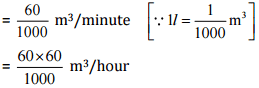∴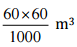water filled in reservoir will take = 1 hour

∴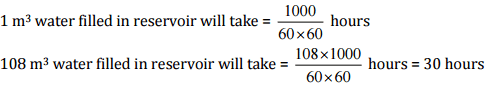It will take 30 hrs. to fill the reservior

The document NCERT Solutions (Ex - 11.3, 11.4) - Mensuration Notes - Class 8 is a part of Class 8 category.
All you need of Class 8 at this link: Class 8Use Code STAYHOME200 and get INR 200 additional OFF Use Coupon Code

Top Courses for Class 8Top Courses for Class 8Track your progress, build streaks, highlight & save important lessons and more!

,

,

,

,

,

,

,

,

,

,

,

,

,

,

,

,

,

,

,

,

,

,

,

,

;